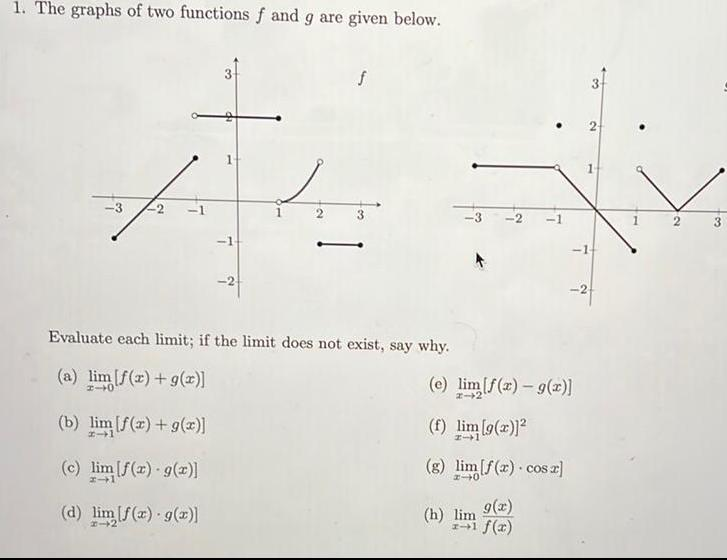Question:

# 1 The graphs of two functions f and g are given below 11

Last updated: 9/16/20231 The graphs of two functions f and g are given below 11 Evaluate each limit if the limit does not exist say why a lim f x g x 40 b lim f x g x c lim f x g x d lim f x g x 14 e lim f x g x f lim 9 2 g lim f x cos z g x h lim f x 2 3